## Ex 4.5 Question 1.

Draw a rough sketch of a quadrilateral PQRS. Draw its diagonals. Name them. Is the meeting point of the diagonals in the interior or exterior of the quadrilateral?

Solution: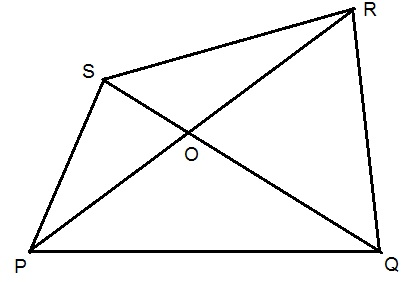## Ex 4.5 Question 2.

2. Draw a rough sketch of a quadrilateral KLMN. State,

(a) two pairs of opposite sides,

(b) two pairs of opposite angles,

(c) two pairs of adjacent sides,

(d) two pairs of adjacent angles.

Solution:(a) Two pairs of opposite sides =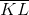,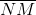and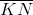,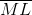(b) Two pairs of opposite angles =  ∠KLM, ∠KNM and ∠LKN, ∠LMN

(c) Two pairs of adjacent sides =,and,or,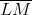and,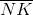(d) Two pairs of adjacent angles = ∠K, ∠L and ∠M, ∠N or ∠K, ∠L, and ∠L, ∠M

error: Content is protected !!